## Excircle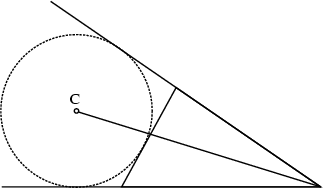Given a Triangle, extend two nonadjacent sides. The Circle tangent to these two lines and to the other side of the Triangle is called an Escribed Circle, or excircle. The Centerof the excircle is called the Excenter and lies on the external Angle Bisector of the opposite Angle. Every Triangle has three excircles, and the Trilinear Coordinates of the Excenters are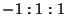,, and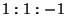. The Radiusof the excircleis called its Exradius.

Given a Triangle with Inradius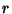, let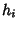be the Altitudes of the excircles, andtheir Radii (the Exradii). Then(Johnson 1929, p. 189).

See also Excenter, Excenter-Excenter Circle, Excentral Triangle, Feuerbach's Theorem, Nagel Point, Triangle Transformation Principle

References

Coxeter, H. S. M. and Greitzer, S. L. Geometry Revisited. Washington, DC: Math. Assoc. Amer., pp. 11-13, 1967.

Johnson, R. A. Modern Geometry: An Elementary Treatise on the Geometry of the Triangle and the Circle. Boston, MA: Houghton Mifflin, pp. 176-177 and 182-194, 1929.